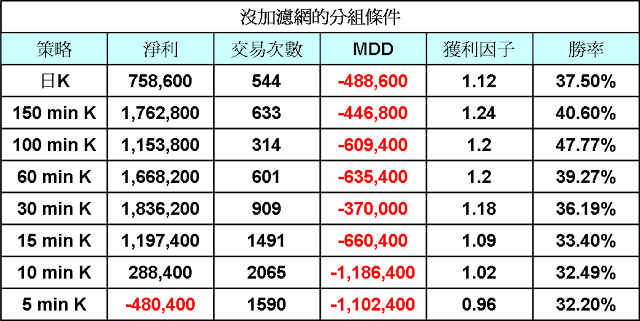## 2013年11月20日 星期三

### 國外知名交易系統 一目均衡表交易模型 Part 2 (程式碼)

Vars: MP(0),IsBalanceDay(false);

ConvertLine = (highest(high,ShortTerm) + Lowest(Low,ShortTerm))/2 ;
BaseLine = (highest(high,MidTerm) + Lowest(Low,MidTerm))/2 ;
AheadLine1 = (ConvertLine + BaseLine)/2 ;
AheadLine2 = (highest(high,LongTerm) + Lowest(Low,LongTerm))/2 ;
BehindLine = Close ;
Cloud = AbsValue(HighCloud-LowCloud) ;
MP = MarketPosition ;

if DAYofMonth(Date) > 14 and DAYofMonth(Date) < 22 and DAYofWeek(Date)= 3 then isBalanceDay = True else isBalanceDay =False ;

{ ---- 進場規則 --------}
Condition1 = ConvertLine Cross over BaseLine ;
Condition2 = ConvertLine Cross under BaseLine ;
Condition3 = BehindLine > Close[Midterm] ;
Condition4 = BehindLine < Close[Midterm] ;
Condition5 = BehindLine > MaxList(HighCloud,LowCloud) ;
Condition6 = BehindLine < MinList(HighCloud,LowCloud) ;
Condition7 = HighCloud > LowCloud ;
Condition8 = HighCloud < LowCloud ;
Condition9 = Close > BaseLine ;
Condition10 = Close < BaseLine ;
Condition11 = Close > ConvertLine ;
Condition12 = Close < ConvertLine ;
Condition13 = ConvertLine > BaseLine ;
Condition14 = ConvertLine < BaseLine ;

{ ----- 條件組合 ---- Condition3 ~6 為核心 -----}
if Type = 1 then Begin
if Condition1 and Condition3 and Condition5 then Buy next bar at Market ;
if Condition2 and Condition4 and Condition6 then Sell next bar at Market ;
end;

if Type = 2 then Begin
if Condition7 and Condition3 and Condition5 then Buy next bar at Market ;
if Condition8 and Condition4 and Condition6 then Sell next bar at Market ;
end;

if Type = 3 then Begin
if Condition9 and Condition3 and Condition5 then Buy next bar at Market ;
if Condition10 and Condition4 and Condition6 then Sell next bar at Market ;
end;

if Type = 4 then Begin
if Condition11 and Condition3 and Condition5 then Buy next bar at Market ;
if Condition12 and Condition4 and Condition6 then Sell next bar at Market ;
end;

if Type = 5 then Begin
if Condition13 and Condition3 and Condition5 then Buy next bar at Market ;
if Condition14 and Condition4 and Condition6 then Sell next bar at Market ;
end;

{----- 停利 and 停損 -----}

if MP > 0 and Low < EntryPrice*(1-TradeStopLoss) then
ExitLong next bar at EntryPrice*(1-TradeStopLoss) stop ;

if MP > 0 and High > EntryPrice*(1+TradeProfit) then
ExitLong next bar at EntryPrice*(1+TradeProfit) stop ;

if MP < 0 and High > EntryPrice*(1+TradeStopLoss) then
ExitShort next bar at EntryPrice*(1+TradeStopLoss) stop ;

if MP < 0 and Low < EntryPrice*(1-TradeProfit) then
ExitShort next bar at EntryPrice*(1-TradeProfit) stop ;

if IsBalanceDay then SetExitOnClose ;{結算日出場}(以 30分鐘為例){ ---- for Filter rule --------}
if Date <> Date then begin
KB = 0 ;
KS = 0 ;
end;

if StdDev(Close,BarNo) <> 0 then
TTXN_Score = (Close - Average(Close,BarNo))/StdDev(Close,BarNo);
TTXN = 10*TTXN_score + 50 ;

{ ----- 濾網設定----- }
inputs:BarNo(10);
Vars:TTXN(0),TTXN_Score(0),KB(0),KS(0) ;

if DataCompression > 1 then Begin
Condition31 = Open > Close and Close > Open ;
Condition32 = Open < Close and Close < Open ;
end else Begin
Condition31 = OpenD(0) > CloseD(1) and Close > OpenD(0) ;{平盤上作多}
Condition32 = OpenD(0) < CloseD(1) and Close < OpenD(0) ;{平盤下作空}
end;

Condition33 = Close > MaxList(HighW(0),HighW(1)) ; {周規則}
Condition34 = Close < MinList(LowW(0),LowW(1)) ;

Condition35 = KB < 1 ; {多單交易次數}
Condition36 = KS < 1 ; {空單交易次數}

Condition37 = TTXN Cross over HighBand ; {T指標}
Condition38 = TTXN Cross under LowBand ;
Condition39 = TTXN > HighBand ;
Condition40 = TTXN < LowBand ;

{ ----- 濾網加入條件組 ----- }

if Type = 6 then Begin
if Condition31 and Condition3 and Condition5 then Buy next bar at Market ;
if Condition32 and Condition4 and Condition6 then Sell next bar at Market ;
end;

if Type = 7 then Begin
if Condition33 and Condition3 and Condition5 then Buy next bar at Market ;
if Condition34 and Condition4 and Condition6 then Sell next bar at Market ;
end;

if Type = 8 then Begin
if Condition35 and Condition3 and Condition5 then Buy next bar at Market ;
if Condition36 and Condition4 and Condition6 then Sell next bar at Market ;
end;

if Type = 9 then Begin
if Condition37 and Condition3 and Condition5 then Buy next bar at Market ;
if Condition38 and Condition4 and Condition6 then Sell next bar at Market ;
end;

if Type = 10 then Begin
if Condition39 and Condition3 and Condition5 then Buy next bar at Market ;
if Condition40 and Condition4 and Condition6 then Sell next bar at Market ;
end;

if MP <= 0 and MP > 0 then KB = 1 ;
if MP >= 0 and MP < 0 then KS = 1 ;1.此部份的測試以限制交易次數與T指標兩種濾網的表現較佳!
2. 30分K與 60分K在這部份測試反而變差是值得再關注的
3.150分K在兩次測試表現都是穩定的狀況或許是較適合的週期

MagicQS015
{-------- 待續 ---------}